# RD Sharma Solutions for Class 8 Maths Chapter 14 Compound Interest Exercise 14.3

In Exercise 14.3 of Chapter 14, we shall discuss how to compute each of the three quantities, i.e., P, T, R when sufficient data is given. RD Sharma Solutions is the best study material, which is developed in accordance with the latest CBSE pattern available. Solutions here are solved and prepared by our faculty team in a step by step format which helps students understand the concept easily, which in turn, helps in scoring good marks in their exams when practised regularly. Students can download the pdf material from the links provided below.

## Download the Pdf of RD Sharma Solutions for Class 8 Maths Exercise 14.3 Chapter 14 Compound Interest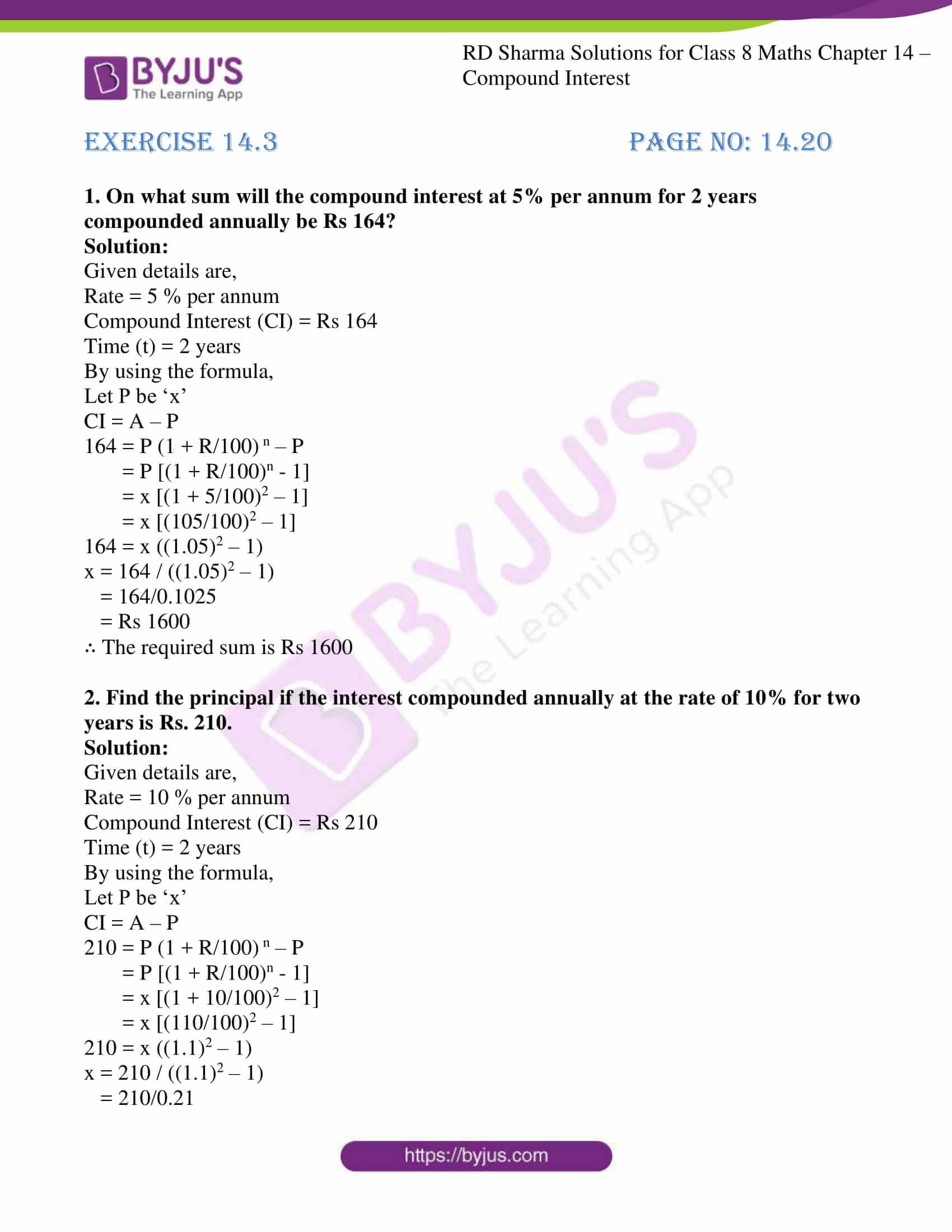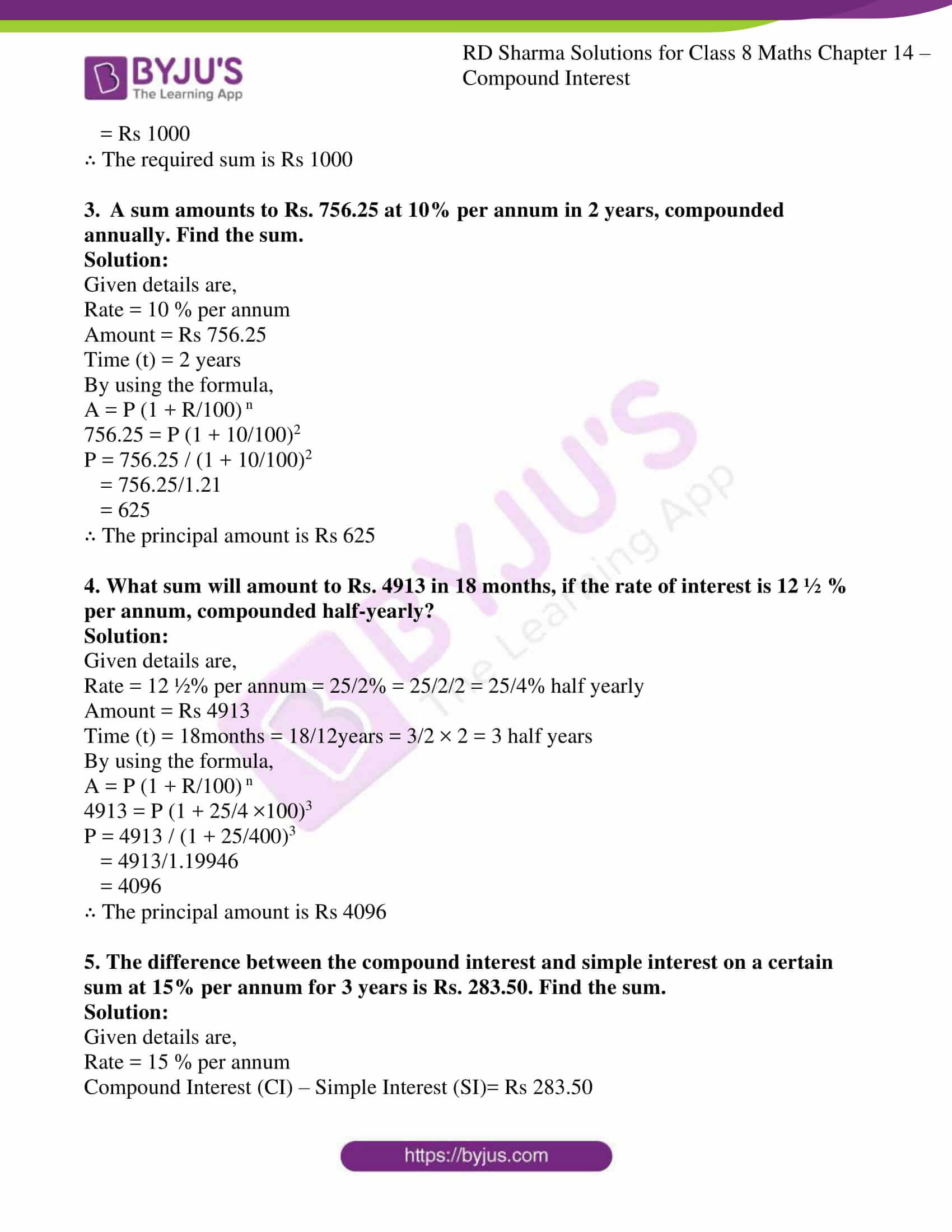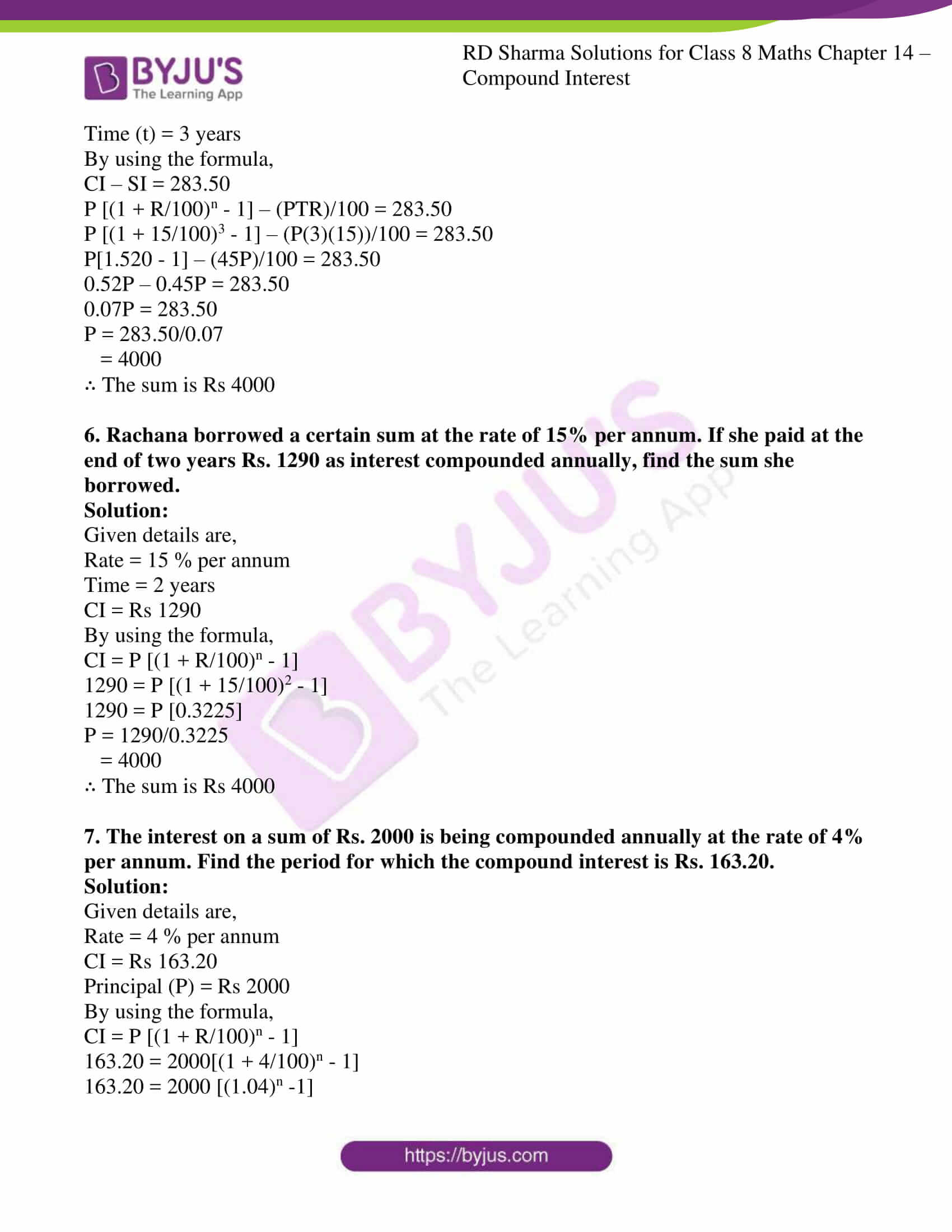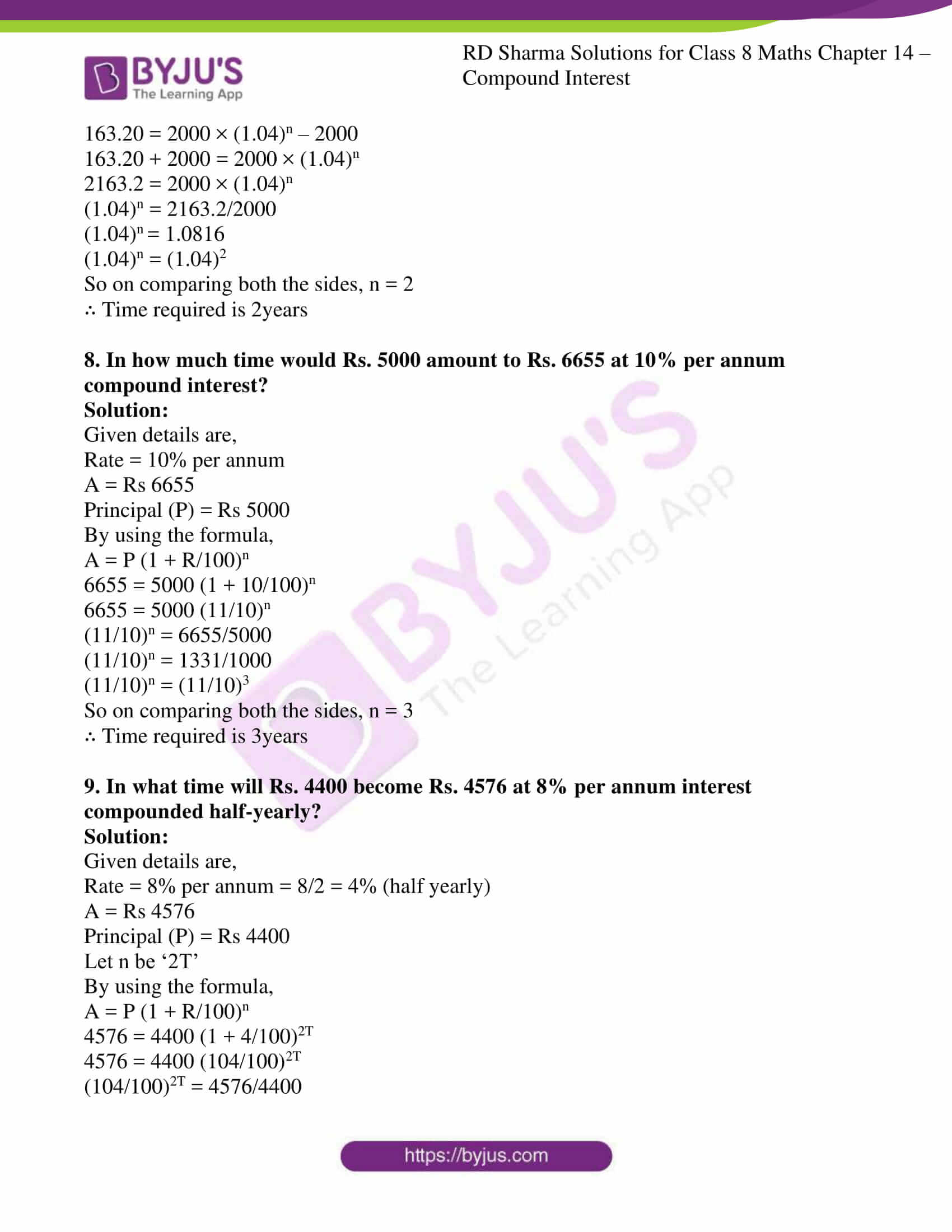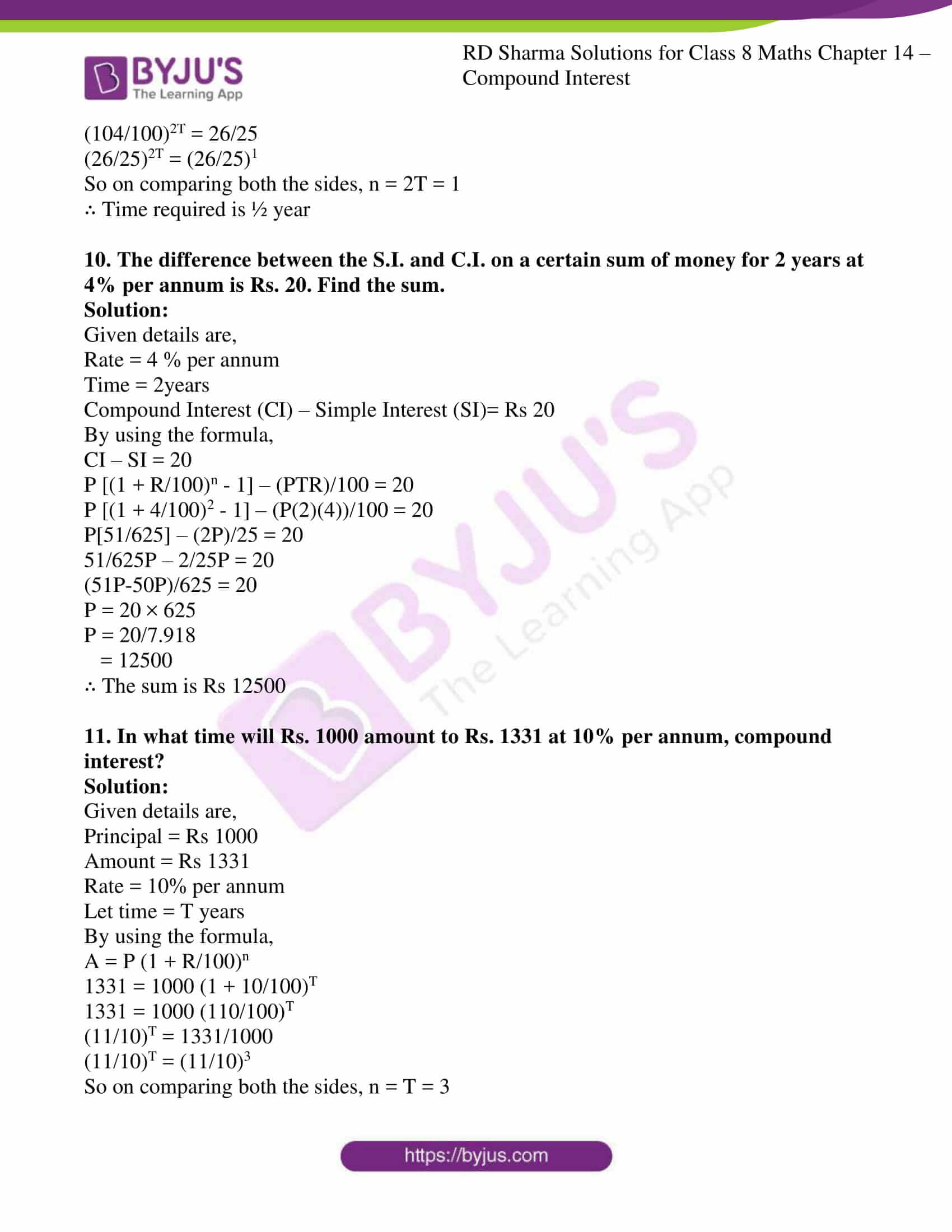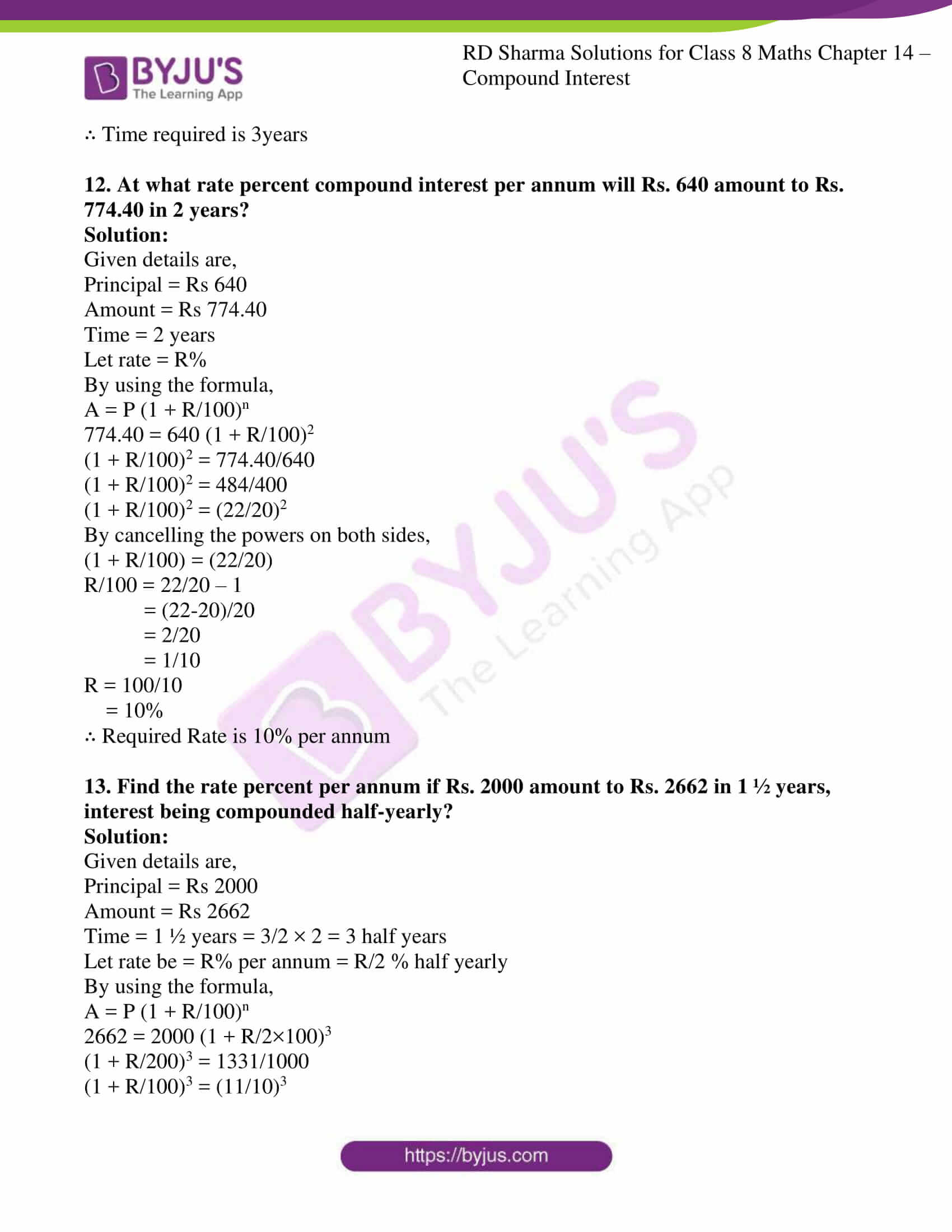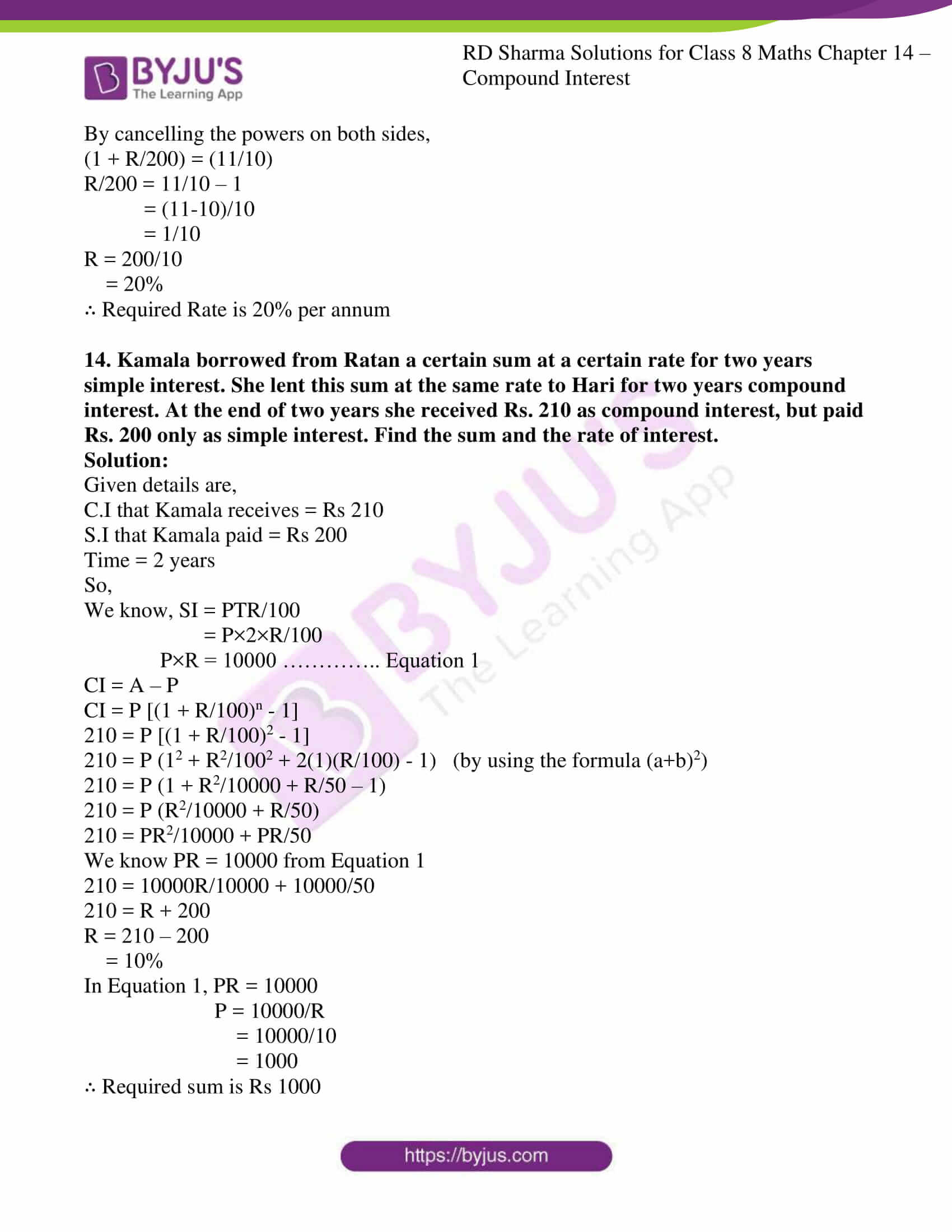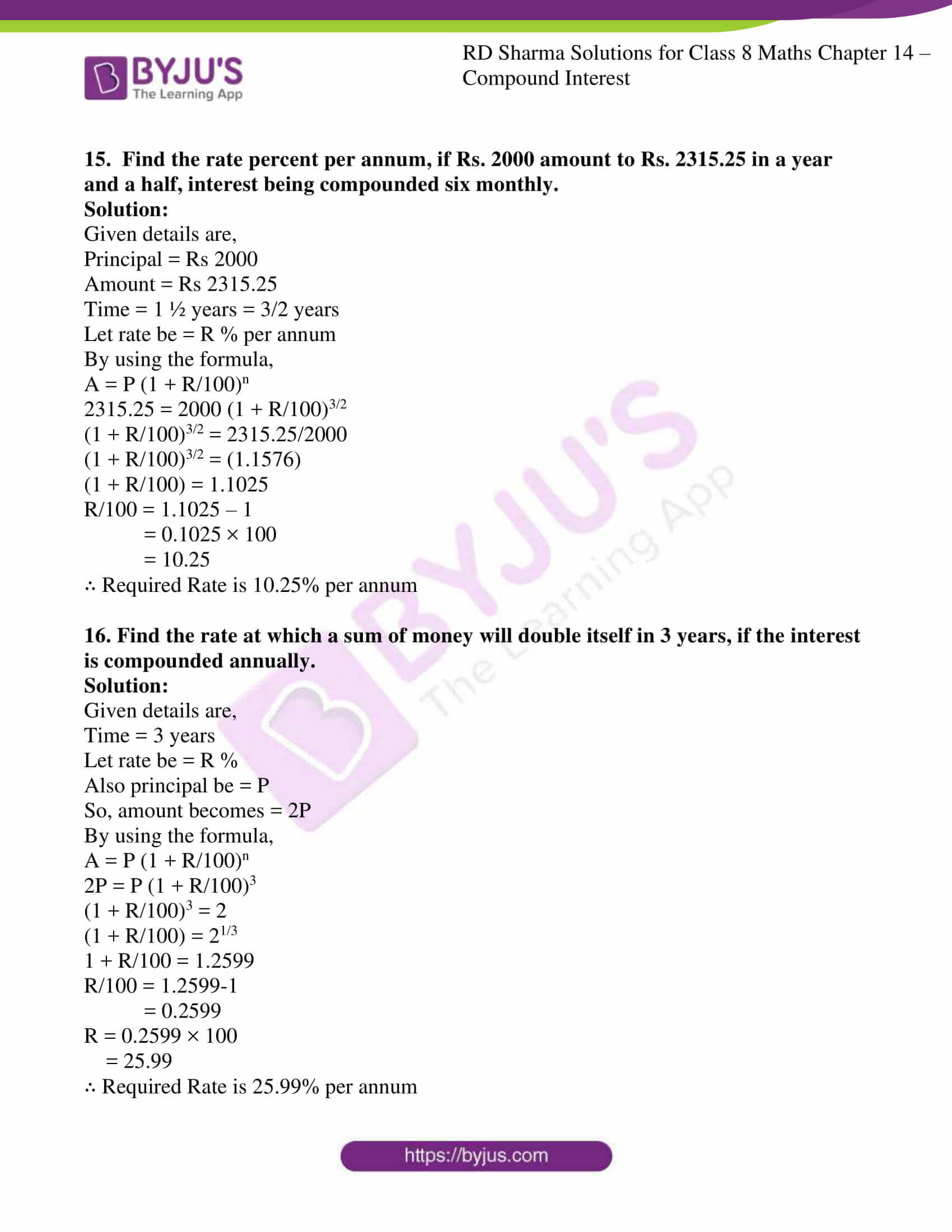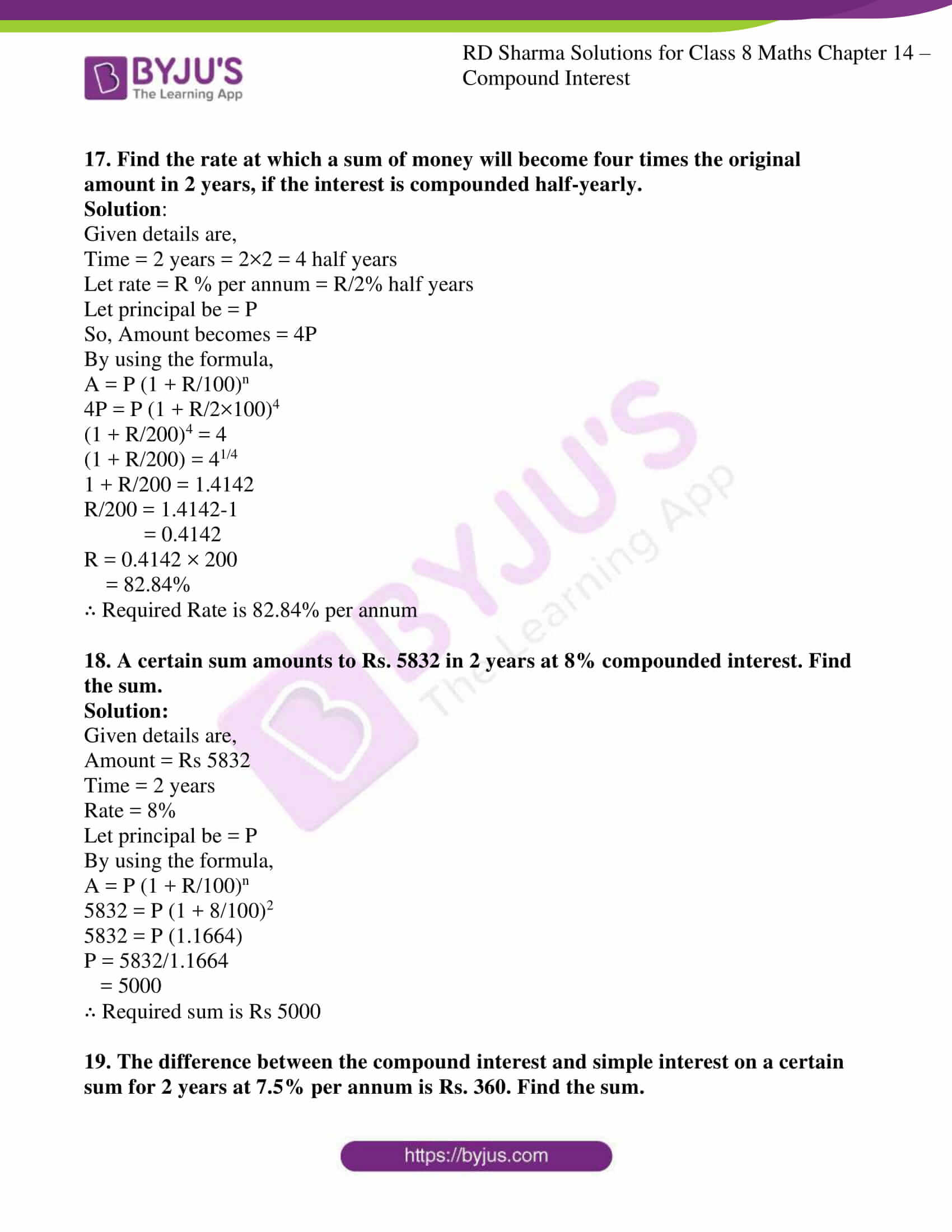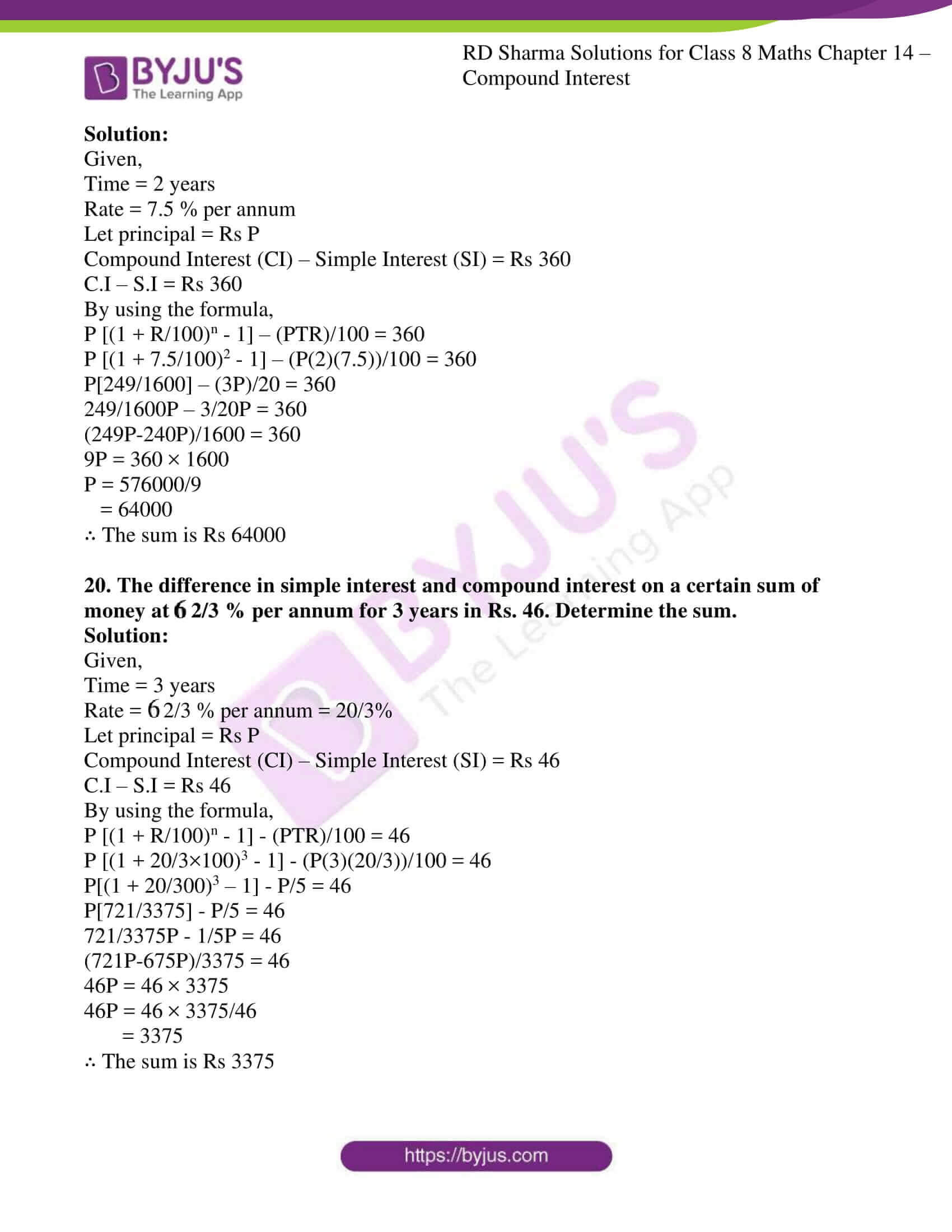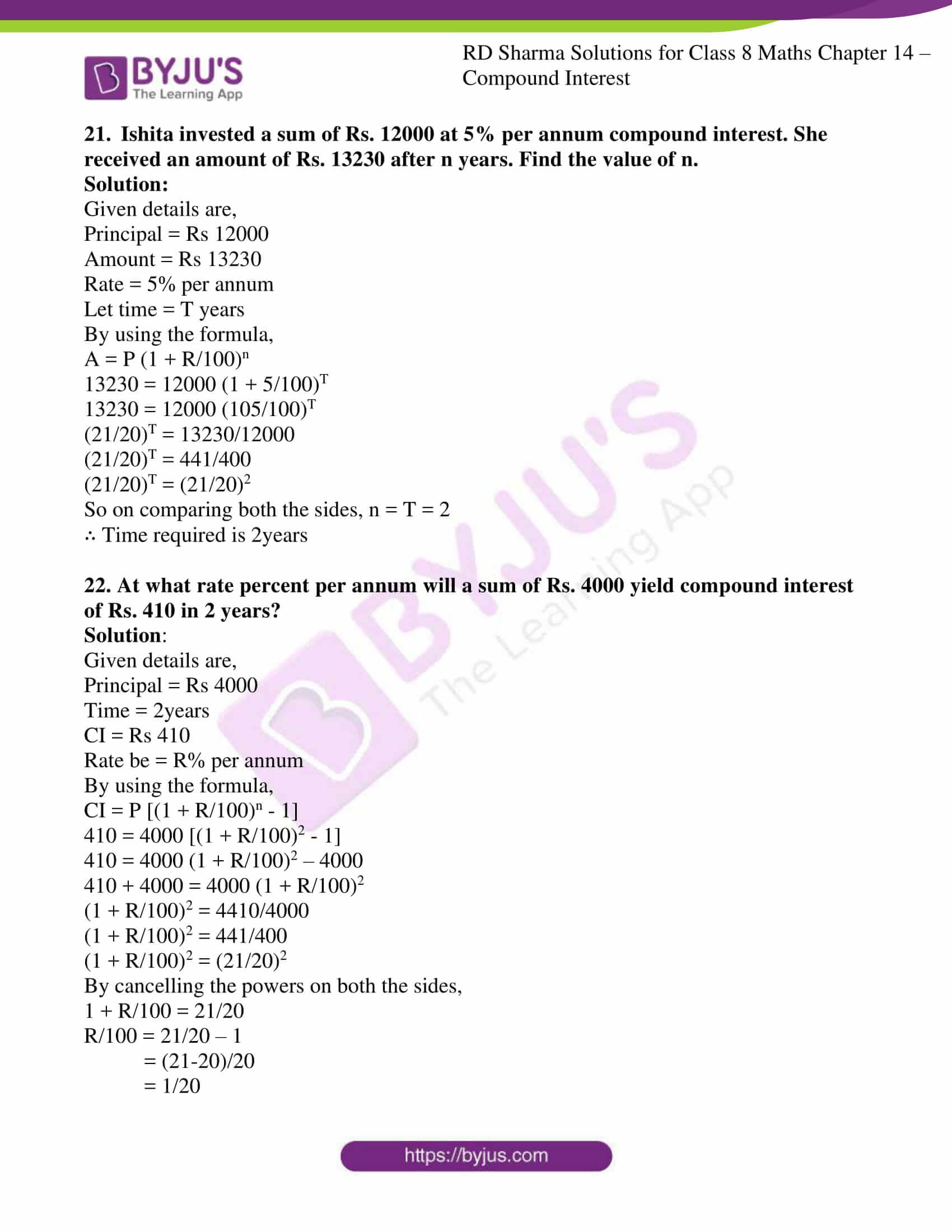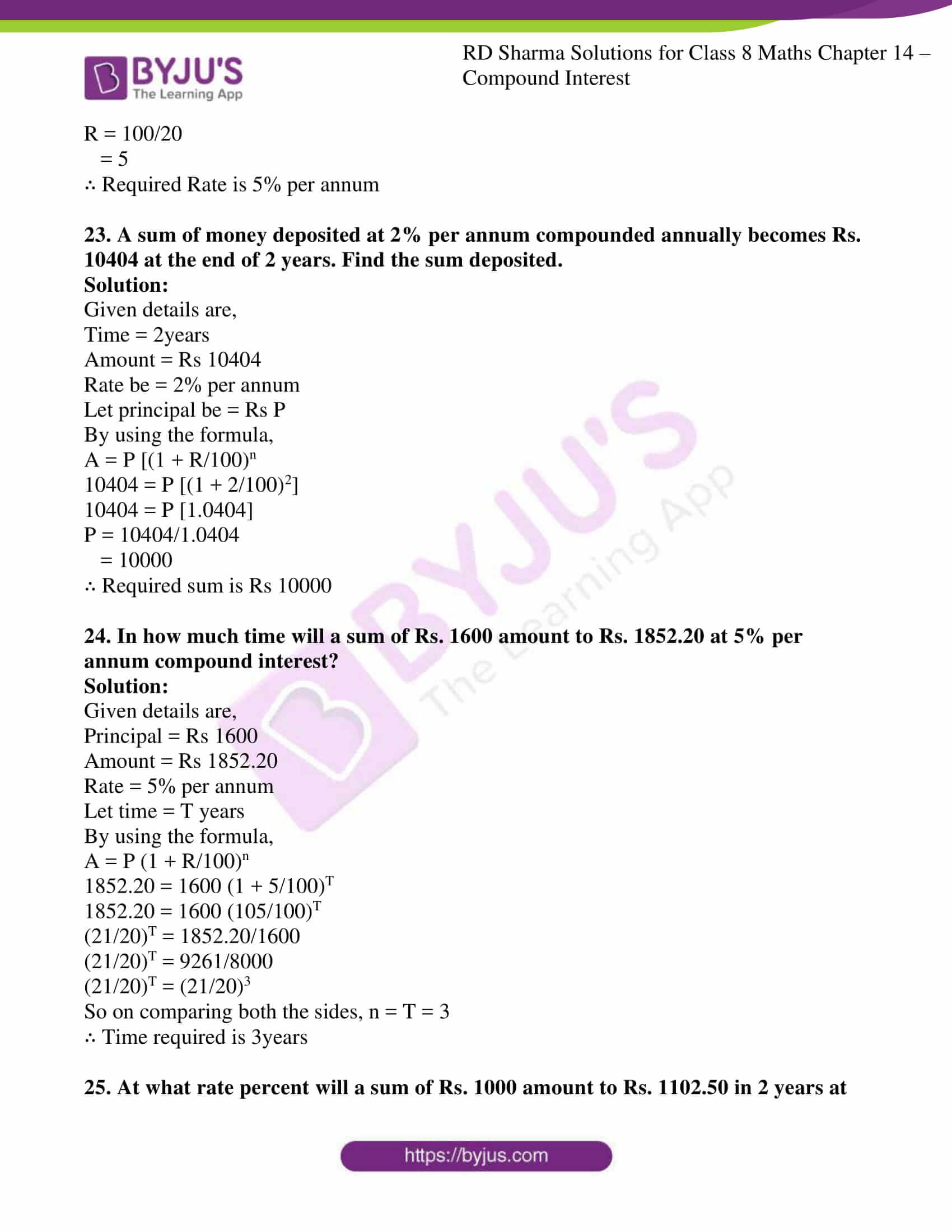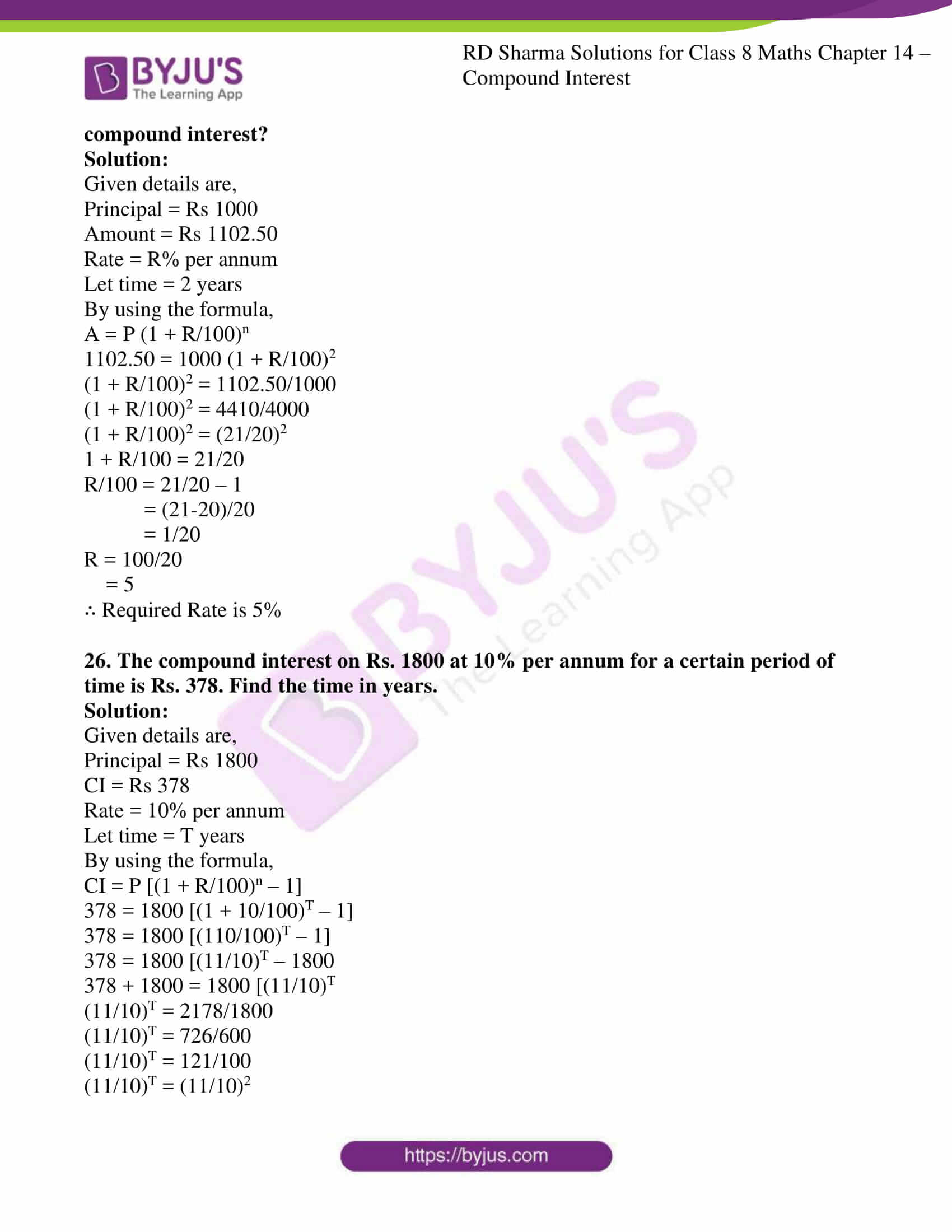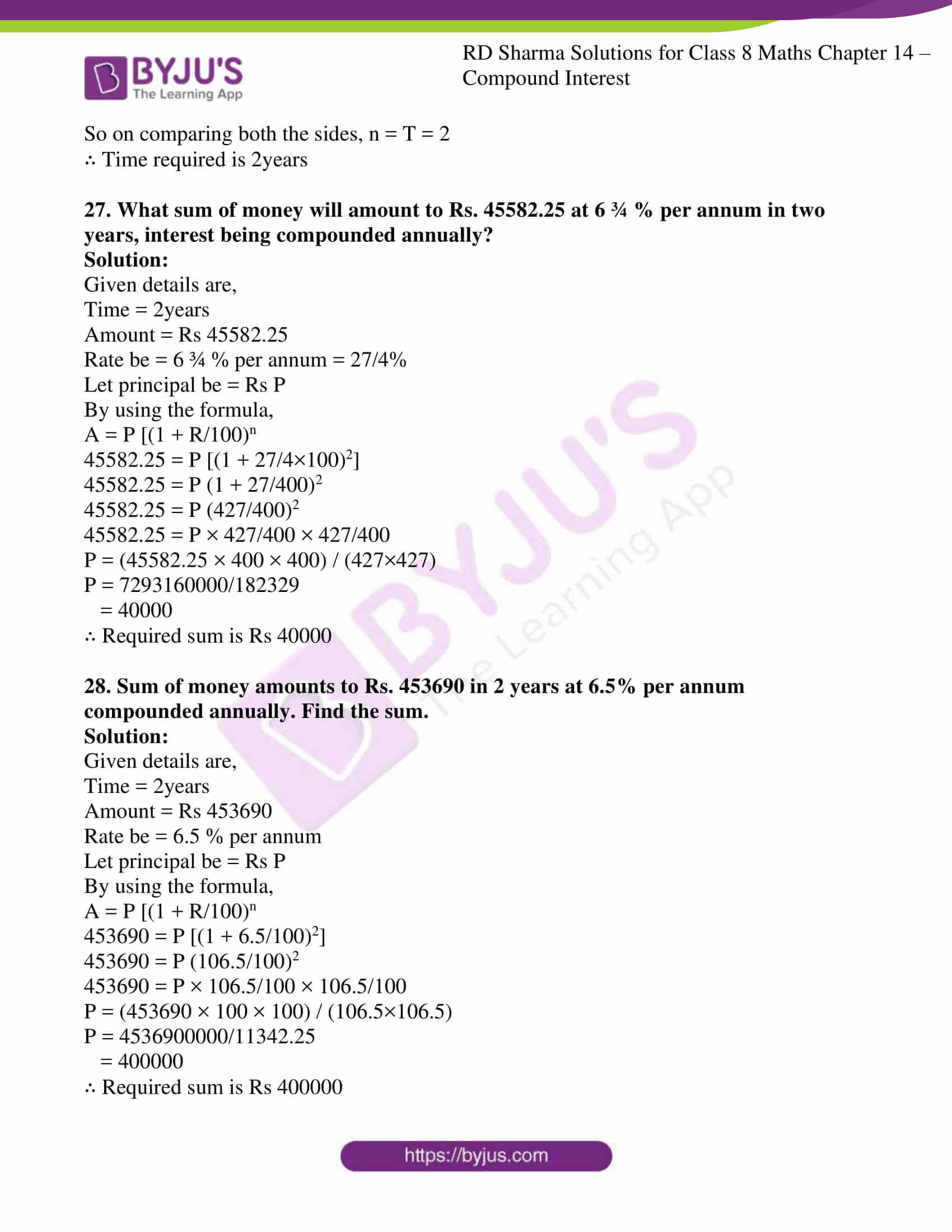### Access answers to Maths RD Sharma Solutions For Class 8 Exercise 14.3 Chapter 14 Compound Interest

1. On what sum will the compound interest at 5% per annum for 2 years compounded annually be Rs 164?

Solution:

Given details are,

Rate = 5 % per annum

Compound Interest (CI) = Rs 164

Time (t) = 2 years

By using the formula,

Let P be ‘x’

CI = A – P

164 = P (1 + R/100) n – P

= P [(1 + R/100)n – 1]

= x [(1 + 5/100)2 – 1]

= x [(105/100)2 – 1]

164 = x ((1.05)2 – 1)

x = 164 / ((1.05)2 – 1)

= 164/0.1025

= Rs 1600

∴ The required sum is Rs 1600

2. Find the principal if the interest compounded annually at the rate of 10% for two years is Rs. 210.

Solution:

Given details are,

Rate = 10 % per annum

Compound Interest (CI) = Rs 210

Time (t) = 2 years

By using the formula,

Let P be ‘x’

CI = A – P

210 = P (1 + R/100) n – P

= P [(1 + R/100)n – 1]

= x [(1 + 10/100)2 – 1]

= x [(110/100)2 – 1]

210 = x ((1.1)2 – 1)

x = 210 / ((1.1)2 – 1)

= 210/0.21

= Rs 1000

∴ The required sum is Rs 1000

3. A sum amounts to Rs. 756.25 at 10% per annum in 2 years, compounded annually. Find the sum.

Solution:

Given details are,

Rate = 10 % per annum

Amount = Rs 756.25

Time (t) = 2 years

By using the formula,

A = P (1 + R/100) n

756.25 = P (1 + 10/100)2

P = 756.25 / (1 + 10/100)2

= 756.25/1.21

= 625

∴ The principal amount is Rs 625

4. What sum will amount to Rs. 4913 in 18 months, if the rate of interest is 12 ½ % per annum, compounded half-yearly?

Solution:

Given details are,

Rate = 12 ½% per annum = 25/2% = 25/2/2 = 25/4% half yearly

Amount = Rs 4913

Time (t) = 18months = 18/12years = 3/2 × 2 = 3 half years

By using the formula,

A = P (1 + R/100) n

4913 = P (1 + 25/4 ×100)3

P = 4913 / (1 + 25/400)3

= 4913/1.19946

= 4096

∴ The principal amount is Rs 4096

5. The difference between the compound interest and simple interest on a certain sum at 15% per annum for 3 years is Rs. 283.50. Find the sum.

Solution:

Given details are,

Rate = 15 % per annum

Compound Interest (CI) – Simple Interest (SI)= Rs 283.50

Time (t) = 3 years

By using the formula,

CI – SI = 283.50

P [(1 + R/100)n – 1] – (PTR)/100 = 283.50

P [(1 + 15/100)3 – 1] – (P(3)(15))/100 = 283.50

P[1.520 – 1] – (45P)/100 = 283.50

0.52P – 0.45P = 283.50

0.07P = 283.50

P = 283.50/0.07

= 4000

∴ The sum is Rs 4000

6. Rachana borrowed a certain sum at the rate of 15% per annum. If she paid at the end of two years Rs. 1290 as interest compounded annually, find the sum she borrowed.

Solution:

Given details are,

Rate = 15 % per annum

Time = 2 years

CI = Rs 1290

By using the formula,

CI = P [(1 + R/100)n – 1]

1290 = P [(1 + 15/100)2 – 1]

1290 = P [0.3225]

P = 1290/0.3225

= 4000

∴ The sum is Rs 4000

7. The interest on a sum of Rs. 2000 is being compounded annually at the rate of 4% per annum. Find the period for which the compound interest is Rs. 163.20.

Solution:

Given details are,

Rate = 4 % per annum

CI = Rs 163.20

Principal (P) = Rs 2000

By using the formula,

CI = P [(1 + R/100)n – 1]

163.20 = 2000[(1 + 4/100)n – 1]

163.20 = 2000 [(1.04)n -1]

163.20 = 2000 × (1.04)n – 2000

163.20 + 2000 = 2000 × (1.04)n

2163.2 = 2000 × (1.04)n

(1.04)n = 2163.2/2000

(1.04)n = 1.0816

(1.04)n = (1.04)2

So on comparing both the sides, n = 2

∴ Time required is 2years

8. In how much time would Rs. 5000 amount to Rs. 6655 at 10% per annum compound interest?

Solution:

Given details are,

Rate = 10% per annum

A = Rs 6655

Principal (P) = Rs 5000

By using the formula,

A = P (1 + R/100)n

6655 = 5000 (1 + 10/100)n

6655 = 5000 (11/10)n

(11/10)n = 6655/5000

(11/10)n = 1331/1000

(11/10)n = (11/10)3

So on comparing both the sides, n = 3

∴ Time required is 3years

9. In what time will Rs. 4400 become Rs. 4576 at 8% per annum interest compounded half-yearly?

Solution:

Given details are,

Rate = 8% per annum = 8/2 = 4% (half yearly)

A = Rs 4576

Principal (P) = Rs 4400

Let n be ‘2T’

By using the formula,

A = P (1 + R/100)n

4576 = 4400 (1 + 4/100)2T

4576 = 4400 (104/100)2T

(104/100)2T = 4576/4400

(104/100)2T = 26/25

(26/25)2T = (26/25)1

So on comparing both the sides, n = 2T = 1

∴ Time required is ½ year

10. The difference between the S.I. and C.I. on a certain sum of money for 2 years at 4% per annum is Rs. 20. Find the sum.

Solution:

Given details are,

Rate = 4 % per annum

Time = 2years

Compound Interest (CI) – Simple Interest (SI)= Rs 20

By using the formula,

CI – SI = 20

P [(1 + R/100)n – 1] – (PTR)/100 = 20

P [(1 + 4/100)2 – 1] – (P(2)(4))/100 = 20

P[51/625] – (2P)/25 = 20

51/625P – 2/25P = 20

(51P-50P)/625 = 20

P = 20 × 625

P = 20/7.918

= 12500

∴ The sum is Rs 12500

11. In what time will Rs. 1000 amount to Rs. 1331 at 10% per annum, compound interest?

Solution:

Given details are,

Principal = Rs 1000

Amount = Rs 1331

Rate = 10% per annum

Let time = T years

By using the formula,

A = P (1 + R/100)n

1331 = 1000 (1 + 10/100)T

1331 = 1000 (110/100)T

(11/10)T = 1331/1000

(11/10)T = (11/10)3

So on comparing both the sides, n = T = 3

∴ Time required is 3years

12. At what rate percent compound interest per annum will Rs. 640 amount to Rs. 774.40 in 2 years?

Solution:

Given details are,

Principal = Rs 640

Amount = Rs 774.40

Time = 2 years

Let rate = R%

By using the formula,

A = P (1 + R/100)n

774.40 = 640 (1 + R/100)2

(1 + R/100)2 = 774.40/640

(1 + R/100)2 = 484/400

(1 + R/100)2 = (22/20)2

By cancelling the powers on both sides,

(1 + R/100) = (22/20)

R/100 = 22/20 – 1

= (22-20)/20

= 2/20

= 1/10

R = 100/10

= 10%

∴ Required Rate is 10% per annum

13. Find the rate percent per annum if Rs. 2000 amount to Rs. 2662 in 1 ½ years, interest being compounded half-yearly?

Solution:

Given details are,

Principal = Rs 2000

Amount = Rs 2662

Time = 1 ½ years = 3/2 × 2 = 3 half years

Let rate be = R% per annum = R/2 % half yearly

By using the formula,

A = P (1 + R/100)n

2662 = 2000 (1 + R/2×100)3

(1 + R/200)3 = 1331/1000

(1 + R/100)3 = (11/10)3

By cancelling the powers on both sides,

(1 + R/200) = (11/10)

R/200 = 11/10 – 1

= (11-10)/10

= 1/10

R = 200/10

= 20%

∴ Required Rate is 20% per annum

14. Kamala borrowed from Ratan a certain sum at a certain rate for two years simple interest. She lent this sum at the same rate to Hari for two years compound interest. At the end of two years she received Rs. 210 as compound interest, but paid Rs. 200 only as simple interest. Find the sum and the rate of interest.

Solution:

Given details are,

C.I that Kamala receives = Rs 210

S.I that Kamala paid = Rs 200

Time = 2 years

So,

We know, SI = PTR/100

= P×2×R/100

P×R = 10000 ………….. Equation 1

CI = A – P

CI = P [(1 + R/100)n – 1]

210 = P [(1 + R/100)2 – 1]

210 = P (12 + R2/1002 + 2(1)(R/100) – 1) (by using the formula (a+b)2)

210 = P (1 + R2/10000 + R/50 – 1)

210 = P (R2/10000 + R/50)

210 = PR2/10000 + PR/50

We know PR = 10000 from Equation 1

210 = 10000R/10000 + 10000/50

210 = R + 200

R = 210 – 200

= 10%

In Equation 1, PR = 10000

P = 10000/R

= 10000/10

= 1000

∴ Required sum is Rs 1000

15. Find the rate percent per annum, if Rs. 2000 amount to Rs. 2315.25 in a year and a half, interest being compounded six monthly.

Solution:

Given details are,

Principal = Rs 2000

Amount = Rs 2315.25

Time = 1 ½ years = 3/2 years

Let rate be = R % per annum

By using the formula,

A = P (1 + R/100)n

2315.25 = 2000 (1 + R/100)3/2

(1 + R/100)3/2 = 2315.25/2000

(1 + R/100)3/2 = (1.1576)

(1 + R/100) = 1.1025

R/100 = 1.1025 – 1

= 0.1025 × 100

= 10.25

∴ Required Rate is 10.25% per annum

16. Find the rate at which a sum of money will double itself in 3 years, if the interest is compounded annually.

Solution:

Given details are,

Time = 3 years

Let rate be = R %

Also principal be = P

So, amount becomes = 2P

By using the formula,

A = P (1 + R/100)n

2P = P (1 + R/100)3

(1 + R/100)3 = 2

(1 + R/100) = 21/3

1 + R/100 = 1.2599

R/100 = 1.2599-1

= 0.2599

R = 0.2599 × 100

= 25.99

∴ Required Rate is 25.99% per annum

17. Find the rate at which a sum of money will become four times the original amount in 2 years, if the interest is compounded half-yearly.

Solution:

Given details are,

Time = 2 years = 2×2 = 4 half years

Let rate = R % per annum = R/2% half years

Let principal be = P

So, Amount becomes = 4P

By using the formula,

A = P (1 + R/100)n

4P = P (1 + R/2×100)4

(1 + R/200)4 = 4

(1 + R/200) = 41/4

1 + R/200 = 1.4142

R/200 = 1.4142-1

= 0.4142

R = 0.4142 × 200

= 82.84%

∴ Required Rate is 82.84% per annum

18. A certain sum amounts to Rs. 5832 in 2 years at 8% compounded interest. Find the sum.

Solution:

Given details are,

Amount = Rs 5832

Time = 2 years

Rate = 8%

Let principal be = P

By using the formula,

A = P (1 + R/100)n

5832 = P (1 + 8/100)2

5832 = P (1.1664)

P = 5832/1.1664

= 5000

∴ Required sum is Rs 5000

19. The difference between the compound interest and simple interest on a certain sum for 2 years at 7.5% per annum is Rs. 360. Find the sum.

Solution:

Given,

Time = 2 years

Rate = 7.5 % per annum

Let principal = Rs P

Compound Interest (CI) – Simple Interest (SI) = Rs 360

C.I – S.I = Rs 360

By using the formula,

P [(1 + R/100)n – 1] – (PTR)/100 = 360

P [(1 + 7.5/100)2 – 1] – (P(2)(7.5))/100 = 360

P[249/1600] – (3P)/20 = 360

249/1600P – 3/20P = 360

(249P-240P)/1600 = 360

9P = 360 × 1600

P = 576000/9

= 64000

∴ The sum is Rs 64000

20. The difference in simple interest and compound interest on a certain sum of money at $$6\frac{2}{3}$$ % per annum for 3 years in Rs. 46. Determine the sum.

Solution:

Given,

Time = 3 years

Rate = $$6\frac{2}{3}$$ % per annum = 20/3%

Let principal = Rs P

Compound Interest (CI) – Simple Interest (SI) = Rs 46

C.I – S.I = Rs 46

By using the formula,

P [(1 + R/100)n – 1] – (PTR)/100 = 46

P [(1 + 20/3×100)3 – 1] – (P(3)(20/3))/100 = 46

P[(1 + 20/300)3 – 1] – P/5 = 46

P[721/3375] – P/5 = 46

721/3375P – 1/5P = 46

(721P-675P)/3375 = 46

46P = 46 × 3375

46P = 46 × 3375/46

= 3375

∴ The sum is Rs 3375

21. Ishita invested a sum of Rs. 12000 at 5% per annum compound interest. She received an amount of Rs. 13230 after n years. Find the value of n.

Solution:

Given details are,

Principal = Rs 12000

Amount = Rs 13230

Rate = 5% per annum

Let time = T years

By using the formula,

A = P (1 + R/100)n

13230 = 12000 (1 + 5/100)T

13230 = 12000 (105/100)T

(21/20)T = 13230/12000

(21/20)T = 441/400

(21/20)T = (21/20)2

So on comparing both the sides, n = T = 2

∴ Time required is 2years

22. At what rate percent per annum will a sum of Rs. 4000 yield compound interest of Rs. 410 in 2 years?

Solution:

Given details are,

Principal = Rs 4000

Time = 2years

CI = Rs 410

Rate be = R% per annum

By using the formula,

CI = P [(1 + R/100)n – 1]

410 = 4000 [(1 + R/100)2 – 1]

410 = 4000 (1 + R/100)2 – 4000

410 + 4000 = 4000 (1 + R/100)2

(1 + R/100)2 = 4410/4000

(1 + R/100)2 = 441/400

(1 + R/100)2 = (21/20)2

By cancelling the powers on both the sides,

1 + R/100 = 21/20

R/100 = 21/20 – 1

= (21-20)/20

= 1/20

R = 100/20

= 5

∴ Required Rate is 5% per annum

23. A sum of money deposited at 2% per annum compounded annually becomes Rs. 10404 at the end of 2 years. Find the sum deposited.

Solution:

Given details are,

Time = 2years

Amount = Rs 10404

Rate be = 2% per annum

Let principal be = Rs P

By using the formula,

A = P [(1 + R/100)n

10404 = P [(1 + 2/100)2]

10404 = P [1.0404]

P = 10404/1.0404

= 10000

∴ Required sum is Rs 10000

24. In how much time will a sum of Rs. 1600 amount to Rs. 1852.20 at 5% per annum compound interest?

Solution:

Given details are,

Principal = Rs 1600

Amount = Rs 1852.20

Rate = 5% per annum

Let time = T years

By using the formula,

A = P (1 + R/100)n

1852.20 = 1600 (1 + 5/100)T

1852.20 = 1600 (105/100)T

(21/20)T = 1852.20/1600

(21/20)T = 9261/8000

(21/20)T = (21/20)3

So on comparing both the sides, n = T = 3

∴ Time required is 3years

25. At what rate percent will a sum of Rs. 1000 amount to Rs. 1102.50 in 2 years at compound interest?

Solution:

Given details are,

Principal = Rs 1000

Amount = Rs 1102.50

Rate = R% per annum

Let time = 2 years

By using the formula,

A = P (1 + R/100)n

1102.50 = 1000 (1 + R/100)2

(1 + R/100)2 = 1102.50/1000

(1 + R/100)2 = 4410/4000

(1 + R/100)2 = (21/20)2

1 + R/100 = 21/20

R/100 = 21/20 – 1

= (21-20)/20

= 1/20

R = 100/20

= 5

∴ Required Rate is 5%

26. The compound interest on Rs. 1800 at 10% per annum for a certain period of time is Rs. 378. Find the time in years.

Solution:

Given details are,

Principal = Rs 1800

CI = Rs 378

Rate = 10% per annum

Let time = T years

By using the formula,

CI = P [(1 + R/100)n – 1]

378 = 1800 [(1 + 10/100)T – 1]

378 = 1800 [(110/100)T – 1]

378 = 1800 [(11/10)T – 1800

378 + 1800 = 1800 [(11/10)T

(11/10)T = 2178/1800

(11/10)T = 726/600

(11/10)T = 121/100

(11/10)T = (11/10)2

So on comparing both the sides, n = T = 2

∴ Time required is 2years

27. What sum of money will amount to Rs. 45582.25 at 6 ¾ % per annum in two years, interest being compounded annually?

Solution:

Given details are,

Time = 2years

Amount = Rs 45582.25

Rate be = 6 ¾ % per annum = 27/4%

Let principal be = Rs P

By using the formula,

A = P [(1 + R/100)n

45582.25 = P [(1 + 27/4×100)2]

45582.25 = P (1 + 27/400)2

45582.25 = P (427/400)2

45582.25 = P × 427/400 × 427/400

P = (45582.25 × 400 × 400) / (427×427)

P = 7293160000/182329

= 40000

∴ Required sum is Rs 40000

28. Sum of money amounts to Rs. 453690 in 2 years at 6.5% per annum compounded annually. Find the sum.

Solution:

Given details are,

Time = 2years

Amount = Rs 453690

Rate be = 6.5 % per annum

Let principal be = Rs P

By using the formula,

A = P [(1 + R/100)n

453690 = P [(1 + 6.5/100)2]

453690 = P (106.5/100)2

453690 = P × 106.5/100 × 106.5/100

P = (453690 × 100 × 100) / (106.5×106.5)

P = 4536900000/11342.25

= 400000

∴ Required sum is Rs 400000